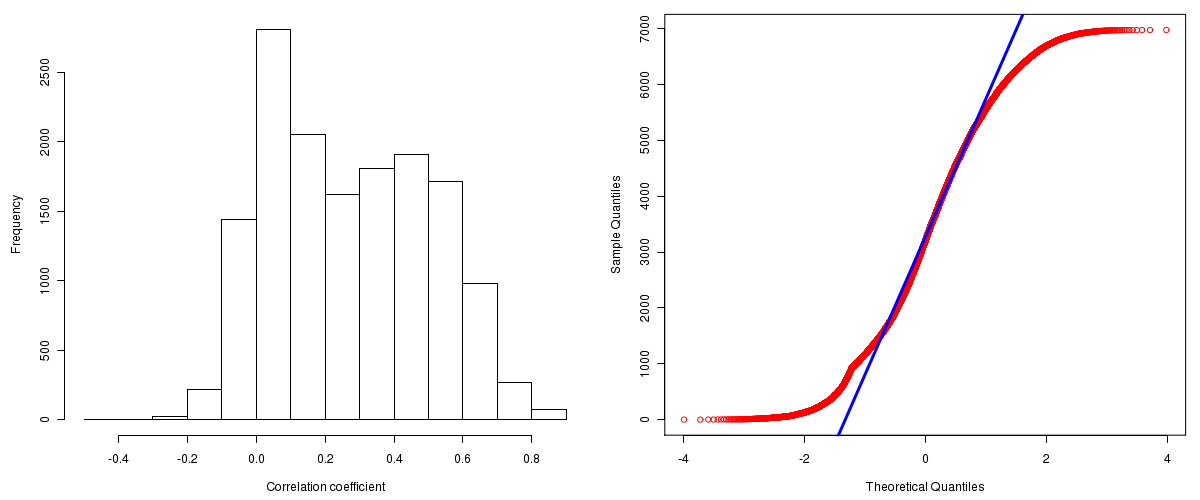Breast Invasive Carcinoma: Correlations between copy number and mRNA expression
Maintained by John Zhang (Dana-Farber Cancer Institute)
Overview
Introduction

A TCGA sample is profiled to detect the copy number variations and expressions of genes. This pipeline attempts to correlate copy number and expression data of genes across samples to determine if the copy number variations also result in differential expressions. This report contains the calculated correlation coefficients based on measurements of genomic copy number (log2) values and intensity of the expressions of the corresponding feature across patients. High positive/low negative correlation coefficients indicate that genomic alterations result in differences in the expressions of mRNA the genomic regions transcribe.

Summary

The correlation coefficients in 10, 20, 30, 40, 50, 60, 70, 80, 90 percentiles are -0.0077, 0.04642, 0.0994, 0.1695, 0.2558, 0.34306, 0.4248, 0.50236, 0.5886, respectively.

Results
Correlation results

Number of genes and samples used for the calculation are shown in Table 1. Figure 1 shows the distribution of calculated correlation coefficients and quantile-quantile plot of the calculated correlation coefficients against a normal distribution. Table 2 shows the top 20 features ordered by the value of correlation coefficients.

Table 1.  Counts of mRNA and number of samples in copy number and expression data sets and common to both

Category Copy number Expression Common
Sample 781 529 500
Genes 29390 17815 15551

Figure 1.  Summary figures. Left: histogram showing the distribution of the calculated correlations across samples for all Genes. Right: QQ plot of the calculated correlations across samples. The QQ plot is used to plot the quantiles of the calculated correlation coefficients against that derived from a normal distribution. Points deviating from the blue line indicate deviation from normality.Table 2.  Get Full Table Top 20 features (defined by the feature column) ranked by correlation coefficients

feature r p-value q-value chrom start end geneid
CLNS1A 0.899 0 0 11 77004847 77026495 1207
STARD3 0.8984 0 0 17 35046938 35073263 10948
LSM1 0.898 0 0 8 38140014 38153183 27257
RPS6KB1 0.8835 0 0 17 55325225 55382569 6198
BRF2 0.8819 0 0 8 37820558 37826569 55290
WHSC1L1 0.879 0 0 8 38251717 38358947 54904
CASC3 0.8768 0 0 17 35550100 35581950 22794
PSMD3 0.8764 0 0 17 35390586 35407738 5709
PPFIA1 0.875 0 0 11 69794471 69908150 8500
MYST3 0.8745 0 0 8 41907420 42028635 7994
RPL19 0.8738 0 0 17 34610062 34614506 6143
SNF8 0.8736 0 0 17 44362457 44377153 11267
ASH2L 0.87 0 0 8 38082223 38116216 9070
UBE2Z 0.8664 0 0 17 44340862 44361417 65264
ATG5 0.8662 0 0 6 106730333 106880361 9474
FADD 0.8645 0 0 11 69726917 69731144 8772
C17orf71 0.858 0 0 17 54642174 54647382 55181
C17orf37 0.8558 0 0 17 35138935 35140314 84299
PROSC 0.8555 0 0 8 37738833 37756444 11212
DDHD2 0.8522 0 0 8 38208264 38238143 23259
Methods & Data
Input

Gene level (TCGA Level III) expression data and copy number data of the corresponding loci derived by using the CNTools package of Bioconductor were used for the calculations. Pearson correlation coefficients were calculated for each pair of genes shared by the two data sets across all the samples that were common.

Correlation across sample

Pairwise correlations between the log2 copy numbers and expressions of each gene across samples were calculated using Pearson correlation.